Solving Matrix-Vector Equations

Doing the same thing a different way, using Mathematica's LinearSolve function: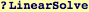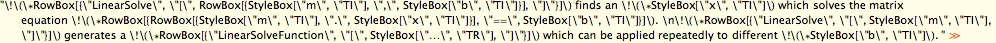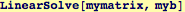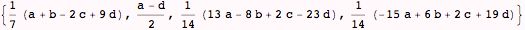And yet another way, based on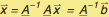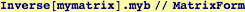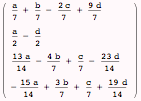And yet even another way, a very efficient LinearSolveFunction can be produced by LinearSolve. This function will operate on any rhs vector of the appropriate length. This would be an efficient way to find the numerical solution to a known matrix, but for many different rhs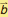.The result can be applied as a function calling a vector :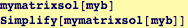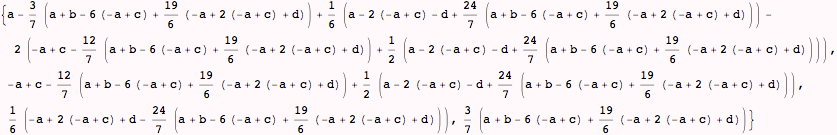Created by Wolfram Mathematica 6.0  (06 September 2007)